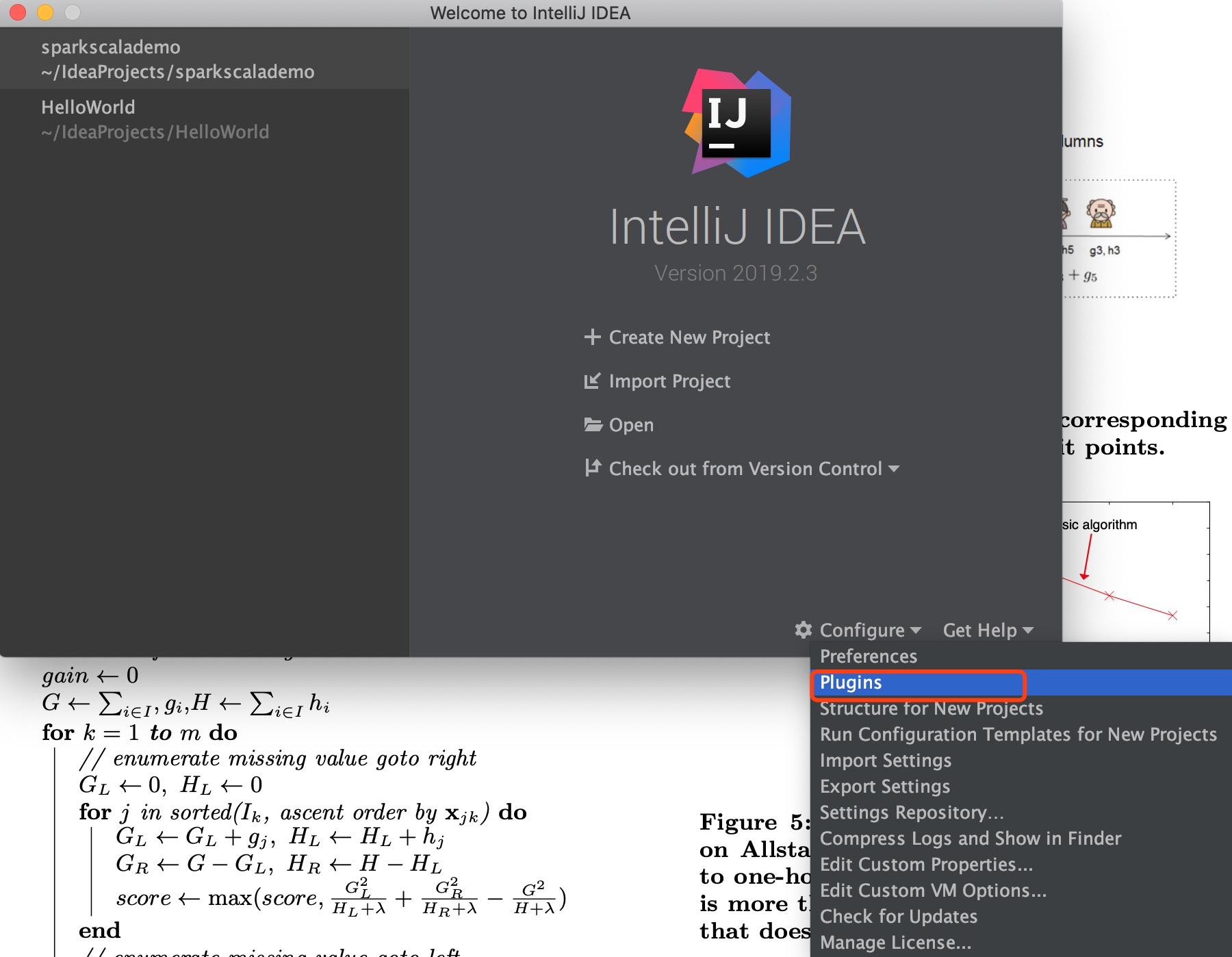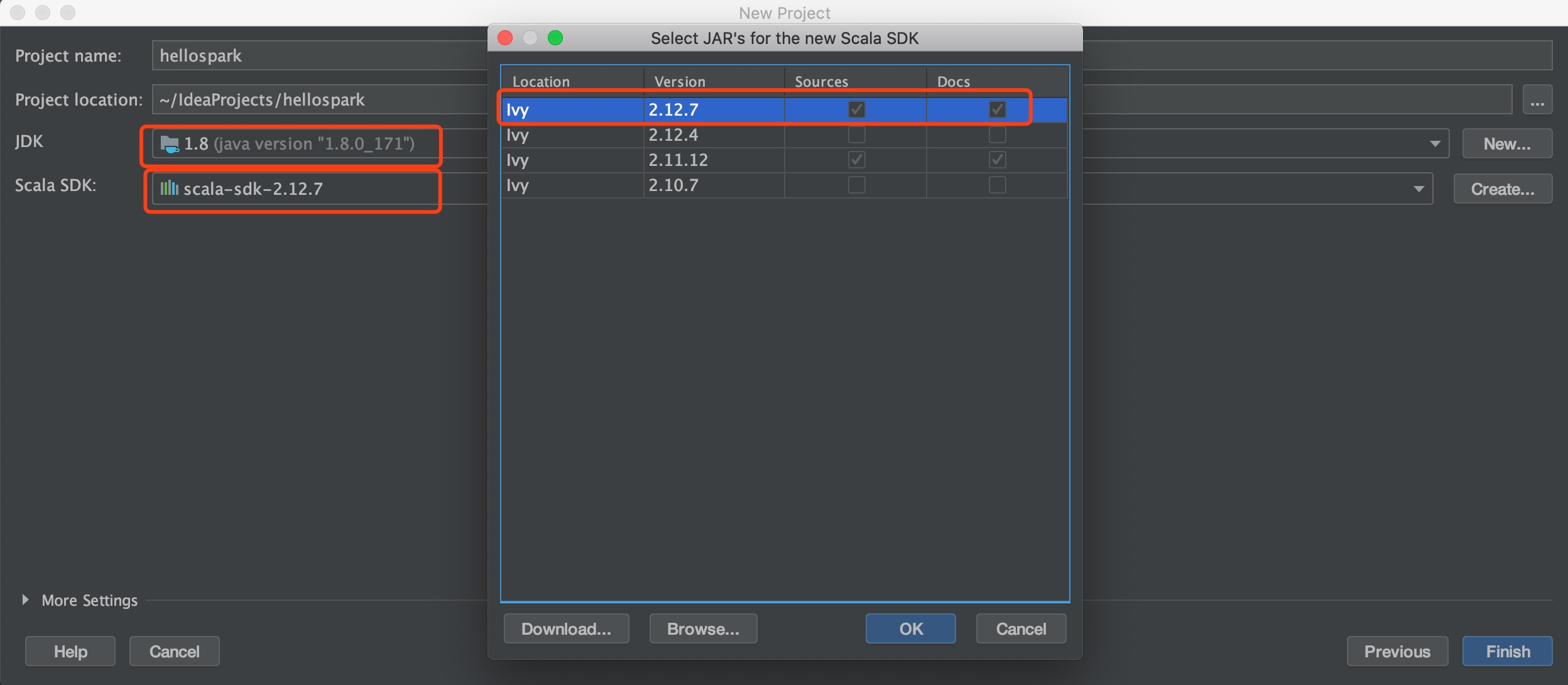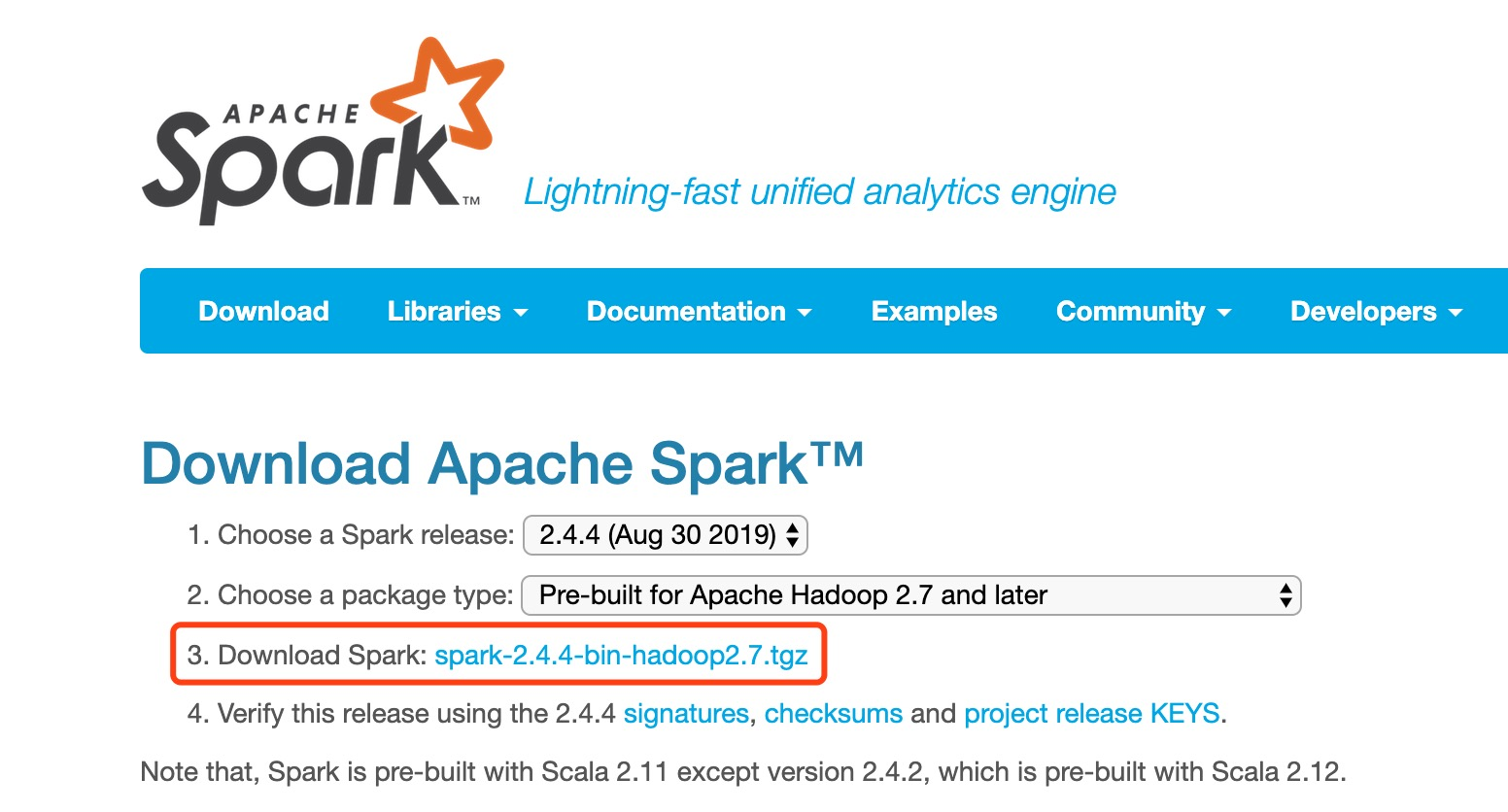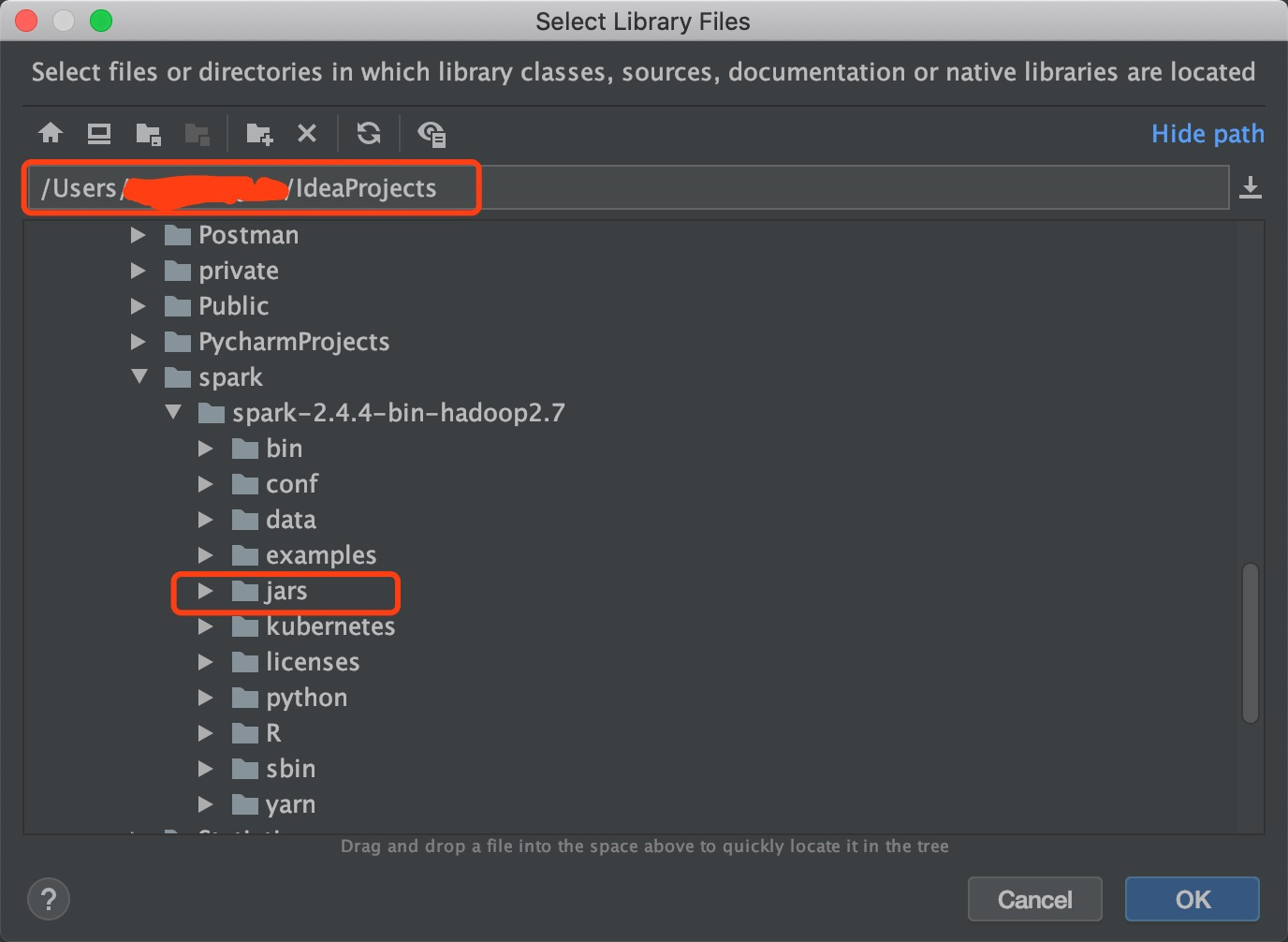## Overview

PySpark是提供了Python语言API接口的Spark，经过我的初步使用，未发现和Scala APISpark有太大差别。且我们服务器上已经配置好了PySpark，正好配合Jupyter notebook使用来进行机器学习离线训练模型。

Spark在我看来就是一个计算工具，用来处理单机计算不了的问题。单机解决不了的问题，无非就是数据太大，内存，磁盘以及处理器不够用，然后把数据拆开给多台机器一起计算。Spark相比较于Hadoop，有一个长处就在于计算。而在机器学习过程当中，从数据预处理到模型训练都需要运算，尤其是数据量比较大的时候。我们尝试对比单机机器学习和分布式机器学习的方式，来加强对Spark的理解。

## 1. 建立Spark会话

from pyspark.sql import SparkSession
from pyspark.sql import functions as F

spark = SparkSession.builder.appName("read data") \ #给任务命名
.master("spark://**.**.**.***:7077") \ #指定spark集群的主节点ip和端口
.config("spark.cores.max", "10") \ #给任务分配CPU
.config("spark.driver.memory", "2G") \ #给每个driver分配内存
.config("spark.executor.memory", "2G") \ #给每个executor分配内存
.config("spark.executor.cores", "2")\ #给每个executor分配CPU
.getOrCreate() #如果当前有那么就复用，如果没有就新建


import pandas as pd


Pandas会把csv文件读取成DataFrame
Spark的处理逻辑也是一样的，会将数据从csv/json/hive/数据库/Hadoop读取为DataFrame，让分析师和算法工程师无缝对接。而语法也是比较简单。

df_spark = spark.read.format("csv").option("header", true").load('hdfs://**.**.**.***:39000/users.csv')


spark.read会返回一个DataFrameReader的实例，通过format指定DataFrameReader读取的数据的格式。

df_spark = spark.read.csv(path='hdfs://**.**.**.***:39000/users.csv', sep=',',  header=True)
df_spark.dtypes


[('name', 'string'),
('sex', 'string'),
('mail', 'string'),
('country', 'string'),
('date', 'string'),
('blood_group', 'string')]


print(df_spark.filter(df.blood_group == 'A+').count())


len(df_single[df_single.blood_group == 'A+'])


country_num = df_spark.groupby(df_spark.country).agg(F.count('*').alias('num')).cache()
print(country_num.collect())


[Row(country='Chad', num=4060), Row(country='Anguilla', num=4015), Row(country='Paraguay', num=4080), Row(country='Macao', num=4071), Row(country='Heard Island and McDonald Islands', num=4042), Row(country='Yemen', num=4102), Row(country='Senegal', num=4188), Row(country='Sweden', num=4092), Row(country='Tokelau', num=4171), Row(country='French Southern Territories', num=4032),
...,
Row(country='Kiribati', num=4058), Row(country='Guyana', num=4134), Row(country='Eritrea', num=4032), Row(country='Jersey', num=3931), Row(country='Philippines', num=4012), Row(country='Tonga', num=3988), Row(country='Norfolk Island', num=4074), Row(country='Djibouti', num=4164), Row(country='Malaysia', num=4138), Row(country='Singapore', num=4087)]


## Overview

TensorFlow 2.0集成了Keras，易用性很高，且Keras之后不再单独更新了，而是作为TensorFlow的一个模块来使用。我们这次就用TensorFlow 2.0中的tf.keras来训练我们的结构化数据。

## 1. 导入特征列表及数据

import numpy as np
import pandas as pd
import sklearn
from sklearn.preprocessing import StandardScaler
import tensorflow as tf
from tensorflow.keras import models, layers, losses, metrics

# 特征列表
my_feature_names = list(name_list.transpose())

# 导入数据


## 2. 数据处理和数据集划分

# 空值填充为0
df_total = df_total.fillna(0)

# 划分数据集
df_train = df_total[df_total.apply_time < '2020-01-21 00:00:00']
df_val = df_total[(df_total.apply_time >= '2020-01-21 00:00:00') & (df_total.apply_time < '2020-02-01 00:00:00')]
df_test = df_total[df_total.apply_time >= '2020-02-01 00:00:00']

# 选取我们需要的数据列
train_x = df_train[my_feature_names]
train_y = df_train['label']
val_x = df_val[my_feature_names]
val_y = df_val['label']
test_x = df_test[my_feature_names]
test_y = df_test['label']

# 数据标准化
scaler = StandardScaler()
train_x = scaler.fit_transform(train_x)
val_x = scaler.transform(val_x)
test_x = scaler.transform(test_x)


## 3. 模型构建

tf.keras.backend.clear_session()
METRICS = [
tf.keras.metrics.AUC(name='auc'),
]

def make_model(metrics = METRICS, output_bias=None):
if output_bias is not None:
output_bias = tf.keras.initializers.Constant(output_bias)
model = tf.keras.Sequential([
layers.Dense(
64, activation='relu',
input_shape=(train_x.shape[-1],)),
layers.Dropout(0.2),
layers.Dense(
128, activation='relu'),
layers.Dropout(0.2),
layers.Dense(
32, activation='relu'),
layers.Dense(1, activation='sigmoid',
bias_initializer=output_bias),
])

model.compile(
loss=losses.BinaryCrossentropy(),
metrics=metrics)

return model
# 设置早停
EPOCHS = 100
BATCH_SIZE = 2000

early_stopping = tf.keras.callbacks.EarlyStopping(
monitor='val_auc',
verbose=1,
patience=20,
mode='max',
restore_best_weights=True)

# 处理不平衡问题
neg = len(train_y) - sum(train_y)
pos = sum(train_y)
total = len(train_y)
weight_for_0 = (1 / neg)*(total)/2.0
weight_for_1 = (1 / pos)*(total)/2.0

class_weight = {0: weight_for_0, 1: weight_for_1}

# 构建模型
model = make_model()
model.summary()


Model: "sequential"
_________________________________________________________________
Layer (type)                 Output Shape              Param #
=================================================================
dense (Dense)                (None, 64)                16384
_________________________________________________________________
dropout (Dropout)            (None, 64)                0
_________________________________________________________________
dense_1 (Dense)              (None, 128)               8320
_________________________________________________________________
dropout_1 (Dropout)          (None, 128)               0
_________________________________________________________________
dense_2 (Dense)              (None, 32)                4128
_________________________________________________________________
dense_3 (Dense)              (None, 1)                 33
=================================================================
Total params: 28,865
Trainable params: 28,865
Non-trainable params: 0


## 4. 模型训练

weighted_history = model.fit(
train_x,
train_y,
batch_size=BATCH_SIZE,
epochs=EPOCHS,
callbacks = [early_stopping],
validation_data=(val_x, val_y),
# 设置类权重
class_weight=class_weight)


Train on 206917 samples, validate on 15830 samples
Epoch 1/100
206917/206917 [==============================] - 3s 12us/sample - loss: 0.6584 - auc: 0.6498 - val_loss: 0.6108 - val_auc: 0.6729
Epoch 2/100
206917/206917 [==============================] - 1s 4us/sample - loss: 0.6305 - auc: 0.6974 - val_loss: 0.6042 - val_auc: 0.6840
Epoch 3/100
206917/206917 [==============================] - 1s 4us/sample - loss: 0.6238 - auc: 0.7075 - val_loss: 0.6018 - val_auc: 0.6895
Epoch 4/100
206917/206917 [==============================] - 1s 4us/sample - loss: 0.6190 - auc: 0.7142 - val_loss: 0.5987 - val_auc: 0.6940
Epoch 5/100
206917/206917 [==============================] - 1s 4us/sample - loss: 0.6157 - auc: 0.7190 - val_loss: 0.5978 - val_auc: 0.6961
Epoch 6/100
206917/206917 [==============================] - 1s 4us/sample - loss: 0.6126 - auc: 0.7230 - val_loss: 0.5957 - val_auc: 0.6989
Epoch 7/100
206917/206917 [==============================] - 1s 4us/sample - loss: 0.6104 - auc: 0.7257 - val_loss: 0.5951 - val_auc: 0.7007
Epoch 8/100
206917/206917 [==============================] - 1s 4us/sample - loss: 0.6082 - auc: 0.7284 - val_loss: 0.5947 - val_auc: 0.7019
Epoch 9/100
206917/206917 [==============================] - 1s 4us/sample - loss: 0.6067 - auc: 0.7301 - val_loss: 0.5937 - val_auc: 0.7034
Epoch 10/100
206917/206917 [==============================] - 1s 4us/sample - loss: 0.6043 - auc: 0.7335 - val_loss: 0.5937 - val_auc: 0.7038
Epoch 11/100
206917/206917 [==============================] - 1s 4us/sample - loss: 0.6035 - auc: 0.7344 - val_loss: 0.5934 - val_auc: 0.7036
Epoch 12/100
206917/206917 [==============================] - 1s 4us/sample - loss: 0.6016 - auc: 0.7365 - val_loss: 0.5924 - val_auc: 0.7046
Epoch 13/100
206917/206917 [==============================] - 1s 4us/sample - loss: 0.6013 - auc: 0.7367 - val_loss: 0.5930 - val_auc: 0.7041
Epoch 14/100
206917/206917 [==============================] - 1s 4us/sample - loss: 0.5996 - auc: 0.7390 - val_loss: 0.5925 - val_auc: 0.7042
Epoch 15/100
206917/206917 [==============================] - 1s 4us/sample - loss: 0.5984 - auc: 0.7403 - val_loss: 0.5930 - val_auc: 0.7045
Epoch 16/100
206917/206917 [==============================] - 1s 4us/sample - loss: 0.5976 - auc: 0.7412 - val_loss: 0.5937 - val_auc: 0.7034
Epoch 17/100
206917/206917 [==============================] - 1s 4us/sample - loss: 0.5961 - auc: 0.7430 - val_loss: 0.5942 - val_auc: 0.7034
Epoch 18/100
206917/206917 [==============================] - 1s 4us/sample - loss: 0.5948 - auc: 0.7444 - val_loss: 0.5946 - val_auc: 0.7027
Epoch 19/100
206917/206917 [==============================] - 1s 4us/sample - loss: 0.5938 - auc: 0.7455 - val_loss: 0.5949 - val_auc: 0.7023
Epoch 20/100
206917/206917 [==============================] - 1s 4us/sample - loss: 0.5924 - auc: 0.7472 - val_loss: 0.5944 - val_auc: 0.7024
Epoch 21/100
206917/206917 [==============================] - 1s 4us/sample - loss: 0.5925 - auc: 0.7471 - val_loss: 0.5953 - val_auc: 0.7028
Epoch 22/100
206917/206917 [==============================] - 1s 4us/sample - loss: 0.5915 - auc: 0.7482 - val_loss: 0.5944 - val_auc: 0.7022
Epoch 23/100
206917/206917 [==============================] - 1s 4us/sample - loss: 0.5906 - auc: 0.7488 - val_loss: 0.5964 - val_auc: 0.7008
Epoch 24/100
206917/206917 [==============================] - 1s 4us/sample - loss: 0.5900 - auc: 0.7496 - val_loss: 0.5947 - val_auc: 0.7025
Epoch 25/100
206917/206917 [==============================] - 1s 4us/sample - loss: 0.5894 - auc: 0.7503 - val_loss: 0.5956 - val_auc: 0.7031
Epoch 26/100
206917/206917 [==============================] - 1s 4us/sample - loss: 0.5882 - auc: 0.7517 - val_loss: 0.5944 - val_auc: 0.7028
Epoch 27/100
206917/206917 [==============================] - 1s 4us/sample - loss: 0.5870 - auc: 0.7532 - val_loss: 0.5975 - val_auc: 0.7001
Epoch 28/100
206917/206917 [==============================] - 1s 4us/sample - loss: 0.5869 - auc: 0.7530 - val_loss: 0.5965 - val_auc: 0.7022
Epoch 29/100
206917/206917 [==============================] - 1s 4us/sample - loss: 0.5861 - auc: 0.7537 - val_loss: 0.5970 - val_auc: 0.7011
Epoch 30/100
206917/206917 [==============================] - 1s 4us/sample - loss: 0.5854 - auc: 0.7543 - val_loss: 0.5960 - val_auc: 0.7015
Epoch 31/100
206917/206917 [==============================] - 1s 4us/sample - loss: 0.5844 - auc: 0.7559 - val_loss: 0.5994 - val_auc: 0.6989
Epoch 32/100
206000/206917 [============================>.] - ETA: 0s - loss: 0.5835 - auc: 0.7568Restoring model weights from the end of the best epoch.
206917/206917 [==============================] - 1s 4us/sample - loss: 0.5836 - auc: 0.7568 - val_loss: 0.5982 - val_auc: 0.6992
Epoch 00032: early stopping


## Overview

• 新用户营销模型、老用户召回模型（支付宝蚂蚁财富基金推荐红包发放等）
• 新用户反欺诈模型
• 新老用户信用额度模型（蚂蚁借呗，腾讯微粒贷，京东金条，微博钱包，美团借钱，各类小贷等）
• 虚拟信用卡贷中风控模型（蚂蚁花呗，京东白条等）
• 贷后催收模型

1. 时间长度
2. 行为类型
3. 统计量

## 2. 特征处理

### 2.1 样本不均衡问题

1. 较多类欠采样
2. 较少类过采样
3. 预测时阈值移动
4. 合成数据（PU learning）
5. 权重缩放或惩罚权重
6. 异常检测的方式（如果好坏样本数量悬殊，例如欺诈检测）
7. 组合/集成学习

### 2.5 特征选择和降维

1. 过滤式
2. 包裹式
3. 嵌入式

1. 无监督降维（PCA等）
2. 有监督降维（LDA等）

• WOE
• IV
• 单特征AUC
• 单特征KS

## 3. 模型构建

### 3.2 机器学习算法选择

1. 样本规模较小，选SVM（不适合稀疏数据）
2. 样本规模适中，XGBoostlightGBMCatBoost
3. 样本规模较大，XGBoostlightGBMCatBoost神经网络

### 3.3 过拟合与欠拟合

#### 3.3.1 过拟合

• 更多训练样本
• 特征选择
• 减小模型规模，修改模型架构
• L1L2正则化
• early stop
• dropout
• 权重衰减
• 加入噪音
• bagging集成

#### 3.3.2 欠拟合

• 增加模型规模，修改模型架构
• 挖掘更好的特征
• 增加特征
• 较小正则化

### 3.4 XGBoost调参训练demo

demo不含任何敏感数据。

# 引入数据处理和模型训练必须的包
import numpy as np
import pandas as pd
import xgboost as xgb

# 加载特征列表
my_feature_names = list(name_list.transpose())
print(len(my_feature_names))

# 加载样本
print(df_total.shape)

# 划分数据集
print(df_total.apply_time.min())
print(df_total.apply_time.max())

df_train = df_total[df_total.apply_time < '2020-01-21 00:00:00']
df_val = df_total[(df_total.apply_time >= '2020-01-21 00:00:00') & (df_total.apply_time < '2020-02-01 00:00:00')]
df_test = df_total[df_total.apply_time >= '2020-02-01 00:00:00']

df_train.label.mean()
df_val.label.mean()
df_test.label.mean()

# 数据处理
train_x = df_train[my_feature_names]
train_y = df_train.get('label')
val_x = df_val[my_feature_names]
val_y = df_val.get('label')
test_x = df_test[my_feature_names]
test_y = df_test.get('label')

class_weight = (1-train_y.mean())/train_y.mean() #负样本(0样本)与正样本(1样本)的数量比
data_train_X = train_x.astype(float)
data_val_X = val_x.astype(float)
data_test_X = test_x.astype(float)

xgb_train = xgb.DMatrix(data_train_X, label=train_y)
xgb_val = xgb.DMatrix(data_val_X, label=val_y)
xgb_test = xgb.DMatrix(data_test_X, label=test_y)


import functools
from bayes_opt import BayesianOptimization

scale_pos_weight_ratio = class_weight

from sklearn.metrics import roc_curve
# 计算KS
def ks_measure(preds, label):
fpr, tpr, thresholds = roc_curve(label, preds)
threshold = None
if threshold is None:
score = np.max(np.abs(tpr - fpr))
else:
idx = np.digitize(threshold, thresholds) - 1
score = np.abs(tpr[idx] - fpr[idx])
return  score

def new_eval_xgb_ks(is_cv, metrics, X_train, y_train, X_validation, y_validation, X_test, y_test,eta, gamma, max_depth, min_child_weight,
subsample,
colsample_bytree, alpha,lambda_s):
'''
我们用验证集效果最好的参数作为最终训练的参数
'''
params = dict()
params['eta'] = eta
params['gamma'] = gamma
params['max_depth'] = int(max_depth)
params['min_child_weight'] = min_child_weight
params['subsample'] = subsample
params['colsample_bytree'] = colsample_bytree
params['alpha'] = alpha
params['lambda'] = lambda_s
params['silent'] = 1
params['seed'] = 696
params['objective'] = 'binary:logistic'
params['scale_pos_weight'] = scale_pos_weight_ratio
params['eval_metric'] = ['auc']
n_estimators = 800
print(params)

# 是否对这组参数做cross validation
if is_cv:
pass
else:
dtr = X_train
dva = X_validation
eval_list = [(dtr,"train"), (dva,"validation")]
clf = xgb.train(params, dtr, n_estimators, eval_list, verbose_eval=30, early_stopping_rounds=20)
y_pred = clf.predict(dva, ntree_limit=clf.best_ntree_limit)
dtest = X_test
y_pred_test = clf.predict(dtest, ntree_limit=clf.best_ntree_limit)

# 用ks选参
if metrics == "ks":
result = ks_measure(y_pred, y_validation)
print("test ks: ", ks_measure(y_pred_test, y_test))
return result

def new_model_selection_bayes_opt(X_train, y_train, X_validation, y_validation, X_test, y_test,classifier='xgb', metrics="ks",
is_cv=False):
num_iter = 150
init_points = 50
if classifier == 'xgb':
eval_xgb_ks_part = functools.partial(new_eval_xgb_ks, is_cv, metrics, X_train, y_train, X_validation, y_validation, X_test, y_test)
classifierBO = BayesianOptimization(eval_xgb_ks_part, {'eta': (0.01, 0.5),
'gamma': (0, 20),
'max_depth': (3, 5),
'min_child_weight': (1, 200),
'subsample': (0.6, 1),
'colsample_bytree': (0.6, 1),
'alpha': (1, 100),
'lambda_s':(1,200)})
classifierBO.maximize(init_points=init_points, n_iter=num_iter)
best_params = classifierBO.max['params']
best_val = classifierBO.max['target']
print(best_val)
print(best_params)
return classifierBO

classifier_max = new_model_selection_bayes_opt(xgb_train, train_y, xgb_val, val_y, xgb_test, test_y, classifier='xgb')


target_bst = classifier_max.max['target']
params_bst = classifier_max.max['params']


# 参数
params = {
'booster': 'gbtree',
'objective': 'binary:logistic',
'alpha': params_bst['alpha'],
'colsample_bytree': params_bst['colsample_bytree'],
'eta': params_bst['eta'],
'gamma': params_bst['gamma'],
'lambda': params_bst['lambda_s'],
'max_depth': int(params_bst['max_depth']),
'min_child_weight': params_bst['min_child_weight'],
'subsample': params_bst['subsample'],
'silent': 1,
'seed': 696,
'scale_pos_weight': scale_pos_weight_ratio,
'eval_metric': ['auc']
}
num_boost = bst_num_boost  # 从输出日志中可以找到最好的树棵数

watchlist = [(xgb_train, 'train'), (xgb_val, 'val'), (xgb_test, 'test')]

# 训练
model = xgb.train(params, xgb_train, num_boost, watchlist)
# 保存模型
model.save_model('result_model.model')  # 用于存储训练出的模型


import numpy as np
import pandas as pd
from sklearn.metrics import roc_curve, auc

def model_evaluation(y, y_pred):
"""
输入真实的y和预测的y的概率
输出ks和auc
"""
result = {}
result['ks']= ks_score(y, y_pred)
result['auc'] = auc_score(y, y_pred)
return result

def ks_score(y, y_pred, threshold=None):
"""
计算KS
"""
fpr, tpr, thresholds = roc_curve(y, y_pred)
if threshold is None:
score = np.max(np.abs(tpr - fpr))
else:
idx = np.digitize(threshold, thresholds) - 1
score = np.abs(tpr[idx] - fpr[idx])
return score

def auc_score(y, y_pred):
"""
计算AUC
"""
fpr, tpr, thresholds = roc_curve(y, y_pred)
roc_auc = auc(fpr, tpr)
return roc_auc

train_pred = model.predict(xgb_train)
val_pred = model.predict(xgb_val)
test_pred = model.predict(xgb_test)

model_evaluation(train_y, train_pred)
model_evaluation(val_y, val_pred)
model_evaluation(test_y, test_pred)


### 3.5 模型预测

model = xgb.Booster()
reg_pred = model.predict(xgb_reg)  # 假设xgb_reg是回归集处理过的数据
online_pred = model.predict(xgb_online)  # 假设xgb_online是上线后的数据集处理过的数据


def psi(bench, target, group=None):
if group is None:
group = int(len(bench)**0.5)
labels_q = np.percentile(bench,[(100.0//group)*i for i in range(group + 1)],interpolation = "nearest")

ben_pct = (pd.cut(bench, bins = np.unique(labels_q),include_lowest = True).value_counts())/len(bench)
target_pct = (pd.cut(target,bins = np.unique(labels_q),include_lowest = True).value_counts())/len(target)
target_pct = target_pct.sort_index()
ben_pct = ben_pct.sort_index()
psi = sum((target_pct - ben_pct)*np.log(target_pct/ben_pct))

return psi


psi(reg_pred, online_pred, group=10) # 10或者20都可以，但是得保证每个bin的数量充足


## 1. IDEA中安装Scala插件## 2. 安装Spark2.4.4## 3. 编写Scala程序

src文件夹上右击，新建一个Scala Class，选择Object，名字叫HelloWorldHelloWorld.scala内容如下:

import org.apache.spark.{SparkConf, SparkContext}
object HelloWorld {
def main(args: Array[String]): Unit={
val conf = new SparkConf()
.setAppName("first spark")
.setMaster("local");

new SparkContext(conf)
.parallelize(List(1,2,3,4,5,6,7,8,9,10)) //传入可迭代对象
.map(x => x*x) // 平方运算
.filter(_%9 == 0) //只要可以被9整除的
.collect()
.foreach(println);
}
}


Exception in thread "main" java.lang.NoSuchMethodError: scala.Predef$.refArrayOps([Ljava/lang/Object;)Lscala/collection/mutable/ArrayOps; at org.apache.spark.util.Utils$.stringToSeq(Utils.scala:2664)
at org.apache.spark.internal.config.ConfigHelpers$.stringToSeq(ConfigBuilder.scala:49) at org.apache.spark.internal.config.TypedConfigBuilder$$anonfuntoSequence1.apply(ConfigBuilder.scala:125) at org.apache.spark.internal.config.TypedConfigBuilder$$anonfun$toSequence$1.apply(ConfigBuilder.scala:125) at org.apache.spark.internal.config.TypedConfigBuilder.createWithDefault(ConfigBuilder.scala:143) at org.apache.spark.internal.config.package$.<init>(package.scala:172)
at org.apache.spark.internal.config.package$.<clinit>(package.scala) at org.apache.spark.SparkConf$.<init>(SparkConf.scala:716)
at org.apache.spark.SparkConf$.<clinit>(SparkConf.scala) at org.apache.spark.SparkConf.set(SparkConf.scala:95) at org.apache.spark.SparkConf.set(SparkConf.scala:84) at org.apache.spark.SparkConf.setAppName(SparkConf.scala:121) at HelloWorld$.main(HelloWorld.scala:5)
at HelloWorld.main(HelloWorld.scala)


Scala SDK版本太新了，我们需要更换版本。

## 4. 设置log输出级别

import org.apache.log4j.{Level, Logger}


Logger.getLogger("org.apache.spark").setLevel(Level.ERROR)


idea设置控制台不打印日志

## 1. 安装python3-psycopg2和libpq-dev

sudo apt-get install python3-psycopg2
sudo apt-get install libpq-dev


pip3 install psycopg2


ERROR: Command errored out with exit status 1:
command: /home/yangbingjiao/anaconda3/bin/python -c 'import sys, setuptools, tokenize; sys.argv = '"'"'/tmp/pip-install-wxj7s4lx/psycopg2/setup.py'"'"'; __file__='"'"'/tmp/pip-install-wxj7s4lx/psycopg2/setup.py'"'"';f=getattr(tokenize, '"'"'open'"'"', open)(__file__);code=f.read().replace('"'"'\r\n'"'"', '"'"'\n'"'"');f.close();exec(compile(code, __file__, '"'"'exec'"'"'))' egg_info --egg-base pip-egg-info
cwd: /tmp/pip-install-wxj7s4lx/psycopg2/
Complete output (23 lines):
running egg_info
creating pip-egg-info/psycopg2.egg-info
writing pip-egg-info/psycopg2.egg-info/PKG-INFO
writing top-level names to pip-egg-info/psycopg2.egg-info/top_level.txt
writing manifest file 'pip-egg-info/psycopg2.egg-info/SOURCES.txt'

pg_config is required to build psycopg2 from source.  Please add the directory
containing pg_config to the \$PATH or specify the full executable path with the
option:

python setup.py build_ext --pg-config /path/to/pg_config build ...

or with the pg_config option in 'setup.cfg'.

If you prefer to avoid building psycopg2 from source, please install the PyPI

For further information please check the 'doc/src/install.rst' file (also at
<http://initd.org/psycopg/docs/install.html>).

----------------------------------------
ERROR: Command errored out with exit status 1: python setup.py egg_info Check the logs for full command output.


## 2.连接PostgreSQL数据库

import psycopg2
import pandas as pd

cur = conn.cursor()
pql = """
SELECT *
FROM database.table
WHERE  create_time >= '2019-09-23' AND create_time < '2019-09-30'
order by create_time
limit 10
"""
cur.execute(pql)
rows = cur.fetchall()
df = pd.DataFrame(rows)
conn.close()


Python3连接PostgreSQL（10.5）数据库